5.2. CMB bispectrum from active models

Different cosmological models differ in their predictions for the statistical distribution of the anisotropies beyond the power spectrum. Future MAP and Planck satellite missions will provide high-precision data allowing definite estimates of non-Gaussian signals in the CMB. It is therefore important to know precisely which are the predictions of all candidate models for the statistical quantities that will be extracted from the new data and identify their specific signatures.

Of the available non-Gaussian statistics, the CMB bispectrum, or the three-point function of Fourier components of the temperature anisotropy, has been perhaps the one best studied in the literature [Gangui & Martin, 2000a]. There are a few cases where the bispectrum may be deduced analytically from the underlying model. The bispectrum can be estimated from simulated CMB sky maps; however, computing a large number of full-sky maps resulting from defects is a much more demanding task. Recently, a precise numerical code to compute it, not using CMB maps and similar to the CMBFAST code (17) for the power spectrum, was developed in [Gangui, Pogosian & Winitzki 2001b]. What follows below is an account of this work.

In a few words, given a suitable model, one can generate a statistical ensemble of realizations of defect matter perturbations. We used a modified Boltzmann code based on CMBFAST to compute the effect of these perturbations on the CMB and found the bispectrum estimator for a given realization of sources. We then performed statistical averaging over the ensemble of realizations to compute the expected CMB bispectrum. (The CMB power spectrum was also obtained as a byproduct.) As a first application, we then computed the expected CMB bispectrum from a model of simulated string networks first introduced by Albrecht et al.  and further developed in [Pogosian & Vachaspati, 1999] and in [Gangui, Pogosian & Winitzki 2001].

We assume that, given a model of active perturbations, such as a string simulation, we can calculate the energy-momentum tensor Tµ(x,) for a particular realization of the sources in a finite spatial volume V0. Here, x is a 3-dimensional coordinate andis the cosmic time. Many simulations are run to obtain an ensemble of random realizations of sources with statistical properties appropriate for the given model. The spatial Fourier decomposition of Tµcan be written as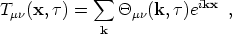(85)

where k are discrete. If V0 is sufficiently large we can approximate the summation by the integral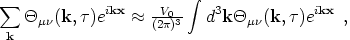(86)

and the corresponding inverse Fourier transform will be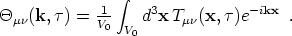(87)

Of course, the final results, such as the CMB power spectrum or bispectrum, do not depend on the choice of V0. To ensure this independence, we shall keep V0 in all expressions where it appears below.

It is conventional to expand the temperature fluctuations over the basis of spherical harmonics,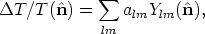(88)

where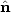is a unit vector. The coefficients alm can be decomposed into Fourier modes,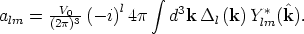(89)

Given the sourcesµ(k,), the quantities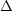l(k) are found by solving linearized Einstein-Boltzmann equations and integrating along the line of sight, using a code similar to CMBFAST [Seljak & Zaldarriaga, 1996]. This standard procedure can be written symbolically as the action of a linear operatorlµ(k) on the source energy-momentum tensor,l(k) =lµ(k)µ(k,), so the third moment ofl(k) is linearly related to the three-point correlator ofµ(k,). Below we consider the quantitiesl(k), corresponding to a set of realizations of active sources, as given. The numerical procedure for computingl(k) was developed in [Albrecht et al. 1997] and in [Pogosian & Vachaspati, 1999].

The third moment of alm, namely <al1 m1 al2 m2 al3 m3> , can be expressed as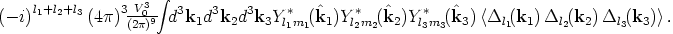(90)

A straightforward numerical evaluation of Eq. (90) from given sourcesl(k) is prohibitively difficult, because it involves too many integrations of oscillating functions. However, we shall be able to reduce the computation to integrations over scalars [a similar method was employed in Komatsu & Spergel, 2001 and in Wang & Kamionkowski, 2000]. Due to homogeneity, the 3-point function vanishes unless the triangle constraint is satisfied,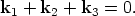(91)

We may write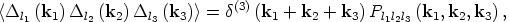(92)

where the three-point function Pl1l2l3(k1, k2, k3) is defined only for values of ki that satisfy Eq. (91). Given the scalar values k1, k2, k3, there is a unique (up to an overall rotation) triplet of directions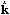i for which the RHS of Eq. (92) does not vanish. The quantity Pl1l2l3(k1, k2, k3) is invariant under an overall rotation of all three vectors ki and therefore may be equivalently represented by a function of scalar values k1, k2, k3, while preserving all angular information. Hence, we can rewrite Eq. (92) as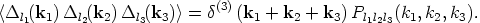(93)

Then, using the simulation volume V0 explicitly, we have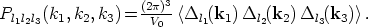(94)

Given an arbitrary direction1 and the magnitudes k1, k2 and k3, the directions2 and3 are specified up to overall rotations by the triangle constraint. Therefore, both sides of Eq. (94) are functions of scalar ki only. The expression on the RHS of Eq. (94) is evaluated numerically by averaging over different realizations of the sources and over permissible directionsi; below we shall give more details of the procedure.

Substituting Eqs. (93) and (94) into (90), Fourier transforming the Dirac delta and using the Rayleigh identity, we can perform all angular integrations analytically and obtain a compact form for the third moment,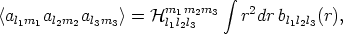(95)

where, denoting the Wigner 3j-symbol by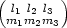, we have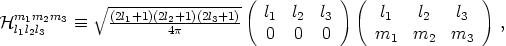(96)

and where we have defined the auxiliary quantities bl1l2l3 using spherical Bessel functions jl,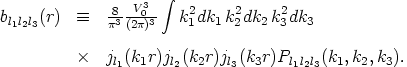(97)

The volume factor V03 contained in this expression is correct: as shown in the next section, each terml includes a factor V0-2/3, while the average quantity Pl1l2l3(k1, k2, k3)V0-3 [cf. Eq. (94)], so that the arbitrary volume V0 of the simulation cancels.

Our proposed numerical procedure therefore consists of computing the RHS of Eq. (95) by evaluating the necessary integrals. For fixed {l1l2l3} , computation of the quantities bl1l2l3(r) is a triple integral over scalar ki defined by Eq. (97); it is followed by a fourth scalar integral over r [Eq. (95)]. We also need to average over many realizations of sources to obtain Pl1l2l3 (k1,k2,k3) . It was not feasible for us to precompute the values Pl1l2l3 (k1, k2, k3) on a grid before integration because of the large volume of data: for each set {l1l2l3} the grid must contain ~ 103 points for each ki. Instead, we precomputel (k) from one realization of sources and evaluate the RHS of Eq. (94) on that data as an estimator of Pl1l2l3 (k1,k2,k3) , averaging over allowed directions ofi. The result is used for integration in Eq. (97).

Because of isotropy and since the allowed sets of directionsi are planar, it is enough to restrict the numerical calculation to directionsi within a fixed two-dimensional plane. This significantly reduces the amount of computations and data storage, sincel (k) only needs to be stored on a two-dimensional grid of k.

In estimating Pl1l2l3 (k1, k2, k3) from Eq. (94), averaging over directions ofi plays a similar role to ensemble averaging over source realizations. Therefore if the number of directions is large enough (we used 720 for cosmic strings), only a moderate number of different source realizations is needed. The main numerical difficulty is the highly oscillating nature of the function bl1l2l3(r). The calculation of the bispectrum for cosmic strings presented in the next Section requires about 20 days of a single-CPU workstation time per realization.

We note that this method is specific for the bispectrum and cannot be applied to compute higher-order correlations. The reason is that higher-order correlations involve configurations of vectors ki that are not described by scalar values ki and not restricted to a plane. For instance, a computation of a 4-point function would involve integration of highly oscillating functions over four vectors ki which is computationally infeasible.

From Eq. (95) we derive the CMB angular bispectruml1l2l3, defined as [Gangui & Martin, 2000b]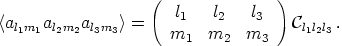(98)

The presence of the 3 j-symbol guarantees that the third moment vanishes unless m1 + m2 + m3 = 0 and the li indices satisfy the triangle rule |li - lj|lkli + lj. Invariance under spatial inversions of the three-point correlation function implies the additional `selection rule' l1 + l2 + l3 = even, in order for the third moment not to vanish. Finally, from this last relation and using standard properties of the 3 j-symbols, it follows that the angular bispectruml1l2l3 is left unchanged under any arbitrary permutation of the indices li.

In what follows we will restrict our calculations to the angular bispectrum Cl1l2l3 in the `diagonal' case, i.e. l1 = l2 = l3 = l. This is a representative case and, in fact, the one most frequently considered in the literature. Plots of the power spectrum are usually done in terms of l(l + 1)Cl which, apart from constant factors, is the contribution to the mean squared anisotropy of temperature fluctuations per unit logarithmic interval of l. In full analogy with this, the relevant quantity to work with in the case of the bispectrum is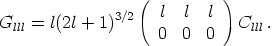(99)

For large values of the multipole index l, Gllll3/2 Clll. Note also what happens with the 3 j-symbols appearing in the definition of the coefficients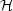l1l2l3m1m2m3: the symbolis absent from the definition of Cl1l2l3, while in Eq. (99) the symbol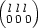is squared. Hence, there are no remnant oscillations due to the alternating sign of.

However, even more important than the value of Clll itself is the relation between the bispectrum and the cosmic variance associated with it. In fact, it is their comparison that tells us about the observability `in principle' of the non-Gaussian signal. The cosmic variance constitutes a theoretical uncertainty for all observable quantities and comes about due to the fact of having just one realization of the stochastic process, in our case, the CMB sky [Scaramella & Vittorio, 1991].

The way to proceed is to employ an estimator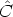l1l2l3 for the bispectrum and compute the variance from it. By choosing an unbiased estimator we ensure it satisfies Cl1l2l3 = <l1l2l3>. However, this condition does not isolate a unique estimator. The proper way to select the best unbiased estimator is to compute the variances of all candidates and choose the one with the smallest value. The estimator with this property was computed in [Gangui & Martin, 2000b] and is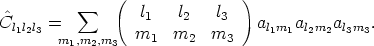(100)

The variance of this estimator, assuming a mildly non-Gaussian distribution, can be expressed in terms of the angular power spectrum Cl as follows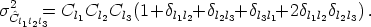(101)

The theoretical signal-to-noise ratio for the bispectrum is then given by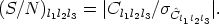(102)

In turn, for the diagonal case l1 = l2 = l3 = l we have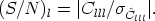(103)

Incorporating all the specifics of the particular experiment, such as sky coverage, angular resolution, etc., will allow us to give an estimate of the particular non-Gaussian signature associated with a given active source and, if observable, indicate the appropriate range of multipole l's where it is best to look for it.

17 http://physics.nyu.edu/matiasz/CMBFAST/cmbfast.html Back.